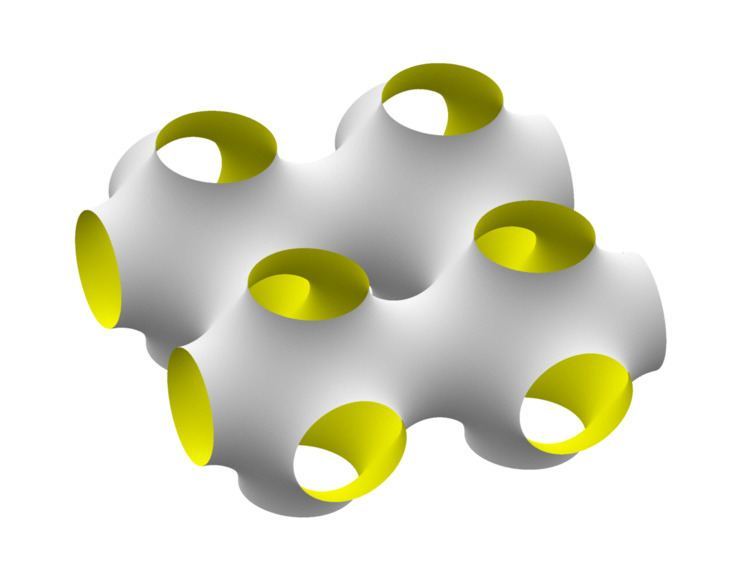Schwarz minimal surface

Updated on
Covid-19In differential geometry, the Schwarz minimal surfaces are periodic minimal surfaces originally described by Hermann Schwarz.

Contents

In the 1880s Schwarz and his student E. R. Neovius described periodic minimal surfaces. They were later named by Alan Schoen in his seminal report that described the gyroid and other triply periodic minimal surfaces.

The surfaces were generated using symmetry arguments: given a solution to Plateau's problem for a polygon, reflections of the surface across the boundary lines also produce valid minimal surfaces that can be continuously joined to the original solution. If a minimal surface meets a plane at right angles, then the mirror image in the plane can also be joined to the surface. Hence given a suitable initial polygon inscribed in a unit cell periodic surfaces can be constructed.

The Schwarz surfaces have topological genus 3, the minimal genus of triply periodic minimal surfaces.

They have been considered as models for periodic nanostructures in block copolymers, electrostatic equipotential surfaces in crystals., and hypothetical negatively curved graphite phases.

Schwarz P ("Primitive")

Schoen named this surface 'primitive' because it has two interwined congruent labyrinths, each with the shape of an inflated tubular version of the simple cubic lattice. While the standard P surface has cubic symmetry the unit cell can be any rectangular box, producing a family of minimal surfaces with the same topology.

It can be approximated by the implicit surface

cos ( x ) + cos ( y ) + cos ( z ) = 0   .

The P surface has been considered for prototyping tissue scaffolds with a high surface-to-volume ratio and porosity.

Schwarz D ("Diamond")

Schoen named this surface 'diamond' because has two intertwined congruent labyrinths, each having the shape of an inflated tubular version of the diamond bond structure. It is sometimes called the F surface in the literature.

It can be approximated by the implicit surface

sin ( x ) sin ( y ) sin ( z ) + sin ( x ) cos ( y ) cos ( z ) + cos ( x ) sin ( y ) cos ( z ) + cos ( x ) cos ( y ) sin ( z ) = 0.

An exact expression exists in terms of elliptic integrals, based on the Weierstrass representation.

Schwarz H ("Hexagonal")

The H surface is similar to a catenoid with a triangular boundary, allowing it to tile space.

Illustrations

• http://www.susqu.edu/brakke/evolver/examples/periodic/periodic.html
• http://www.indiana.edu/~minimal/archive/Triply/genus3.html
• http://www.thphys.uni-heidelberg.de/~biophys/index.php?lang=e&n1=research_tpms
• http://homepages.ulb.ac.be/~morahman/gallery/schwartz.html
• Schwarz minimal surface Wikipedia

Topics